Excel学习网-公益型的EXCEL在线学习网站，助您轻松办公！

# Excel多条件求和SUMIFS函数公式，4种进阶用法

2020年09月21日 18:55 发布来源：Excel学习网

在工作中，多条件求和也是经常需要使用的公式，今天我们来学习SUMIFS函数的使用技巧

1、SUMIFS正常用法

SUMIFS(求和区域，条件区域一，条件一，条件区域二，条件二...)、

=SUMIFS(D:D,C:C,F1)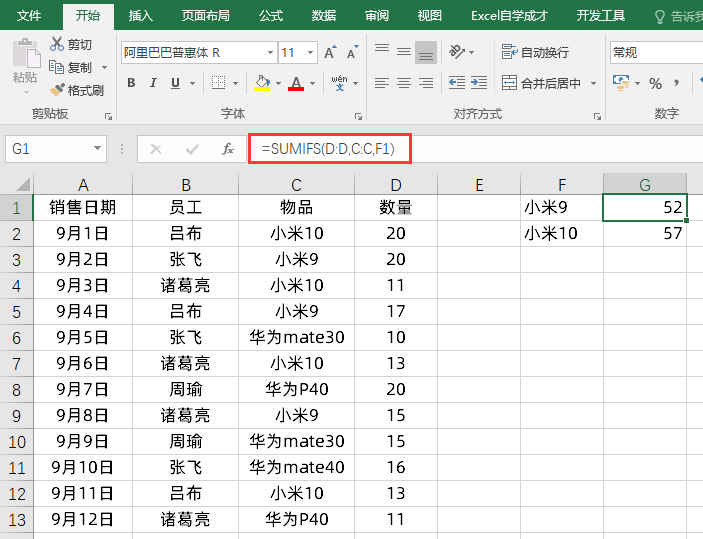=SUMIFS(D:D,C:C,G1,B:B,F1)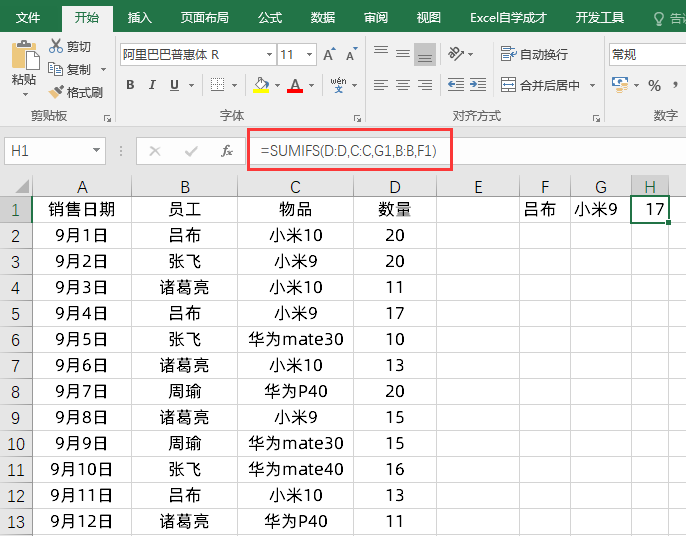2、SUMIFS+通配符

=SUMIFS(D:D,C:C,G1&"*",B:B,F1)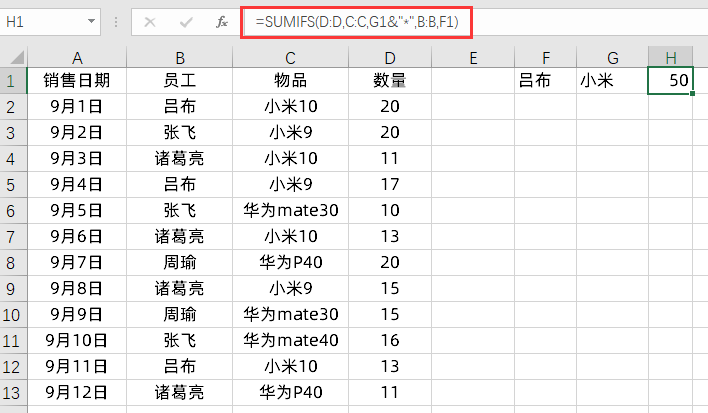3、SUMIFS+时间符运算

=SUMIFS(D:D,A:A,">=9-7")

=SUMIFS(D:D,A:A,">=9/7")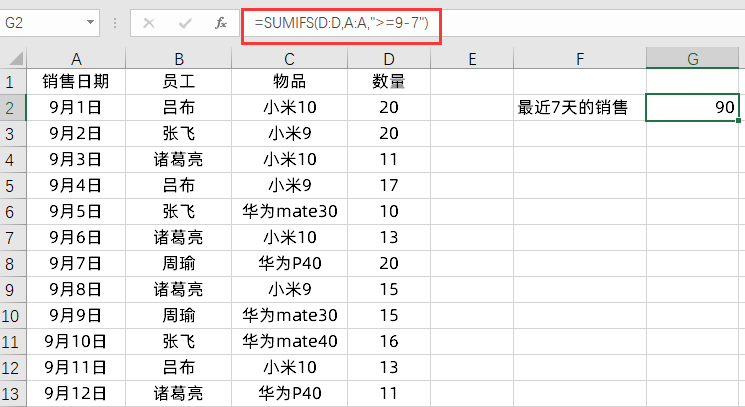=SUMIFS(D:D,A:A,">="&TODAY()-7)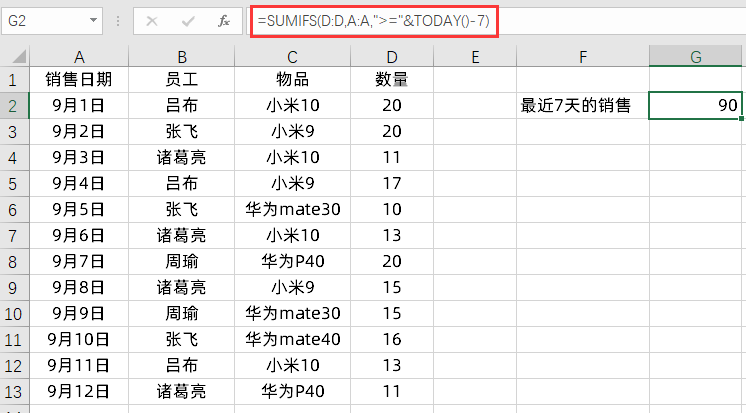4、SUMIFS多个值求和

=SUMPRODUCT(SUMIFS(D:D,C:C,{"小米10","华为mate40"}))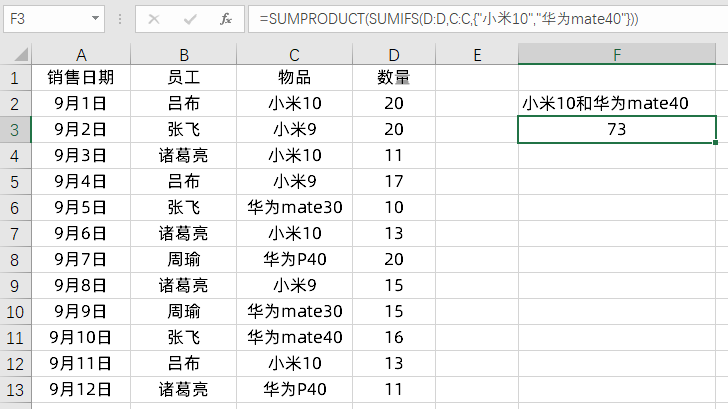Excel优秀工具推荐：